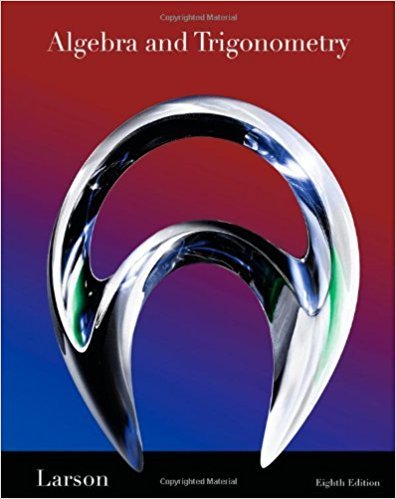×
×

# Solutions for Chapter 4.4: Translations of Conics## Full solutions for Algebra and Trigonometry | 8th Edition

ISBN: 9781439048474Solutions for Chapter 4.4: Translations of Conics

Solutions for Chapter 4.4
4 5 0 353 Reviews
16
2
##### ISBN: 9781439048474

This textbook survival guide was created for the textbook: Algebra and Trigonometry, edition: 8. This expansive textbook survival guide covers the following chapters and their solutions. Since 92 problems in chapter 4.4: Translations of Conics have been answered, more than 47231 students have viewed full step-by-step solutions from this chapter. Algebra and Trigonometry was written by and is associated to the ISBN: 9781439048474. Chapter 4.4: Translations of Conics includes 92 full step-by-step solutions.

Key Math Terms and definitions covered in this textbook
• Associative Law (AB)C = A(BC).

Parentheses can be removed to leave ABC.

• Back substitution.

Upper triangular systems are solved in reverse order Xn to Xl.

• Cayley-Hamilton Theorem.

peA) = det(A - AI) has peA) = zero matrix.

• Cofactor Cij.

Remove row i and column j; multiply the determinant by (-I)i + j •

• Complete solution x = x p + Xn to Ax = b.

(Particular x p) + (x n in nullspace).

• Condition number

cond(A) = c(A) = IIAIlIIA-III = amaxlamin. In Ax = b, the relative change Ilox III Ilx II is less than cond(A) times the relative change Ilob III lib II· Condition numbers measure the sensitivity of the output to change in the input.

• Cyclic shift

S. Permutation with S21 = 1, S32 = 1, ... , finally SIn = 1. Its eigenvalues are the nth roots e2lrik/n of 1; eigenvectors are columns of the Fourier matrix F.

• Dot product = Inner product x T y = XI Y 1 + ... + Xn Yn.

Complex dot product is x T Y . Perpendicular vectors have x T y = O. (AB)ij = (row i of A)T(column j of B).

• Exponential eAt = I + At + (At)2 12! + ...

has derivative AeAt; eAt u(O) solves u' = Au.

• Free variable Xi.

Column i has no pivot in elimination. We can give the n - r free variables any values, then Ax = b determines the r pivot variables (if solvable!).

• Jordan form 1 = M- 1 AM.

If A has s independent eigenvectors, its "generalized" eigenvector matrix M gives 1 = diag(lt, ... , 1s). The block his Akh +Nk where Nk has 1 's on diagonall. Each block has one eigenvalue Ak and one eigenvector.

• Kirchhoff's Laws.

Current Law: net current (in minus out) is zero at each node. Voltage Law: Potential differences (voltage drops) add to zero around any closed loop.

• Normal matrix.

If N NT = NT N, then N has orthonormal (complex) eigenvectors.

• Nullspace N (A)

= All solutions to Ax = O. Dimension n - r = (# columns) - rank.

• Orthogonal matrix Q.

Square matrix with orthonormal columns, so QT = Q-l. Preserves length and angles, IIQxll = IIxll and (QX)T(Qy) = xTy. AlllAI = 1, with orthogonal eigenvectors. Examples: Rotation, reflection, permutation.

• Orthogonal subspaces.

Every v in V is orthogonal to every w in W.

• Particular solution x p.

Any solution to Ax = b; often x p has free variables = o.

• Projection p = a(aTblaTa) onto the line through a.

P = aaT laTa has rank l.

• Reduced row echelon form R = rref(A).

Pivots = 1; zeros above and below pivots; the r nonzero rows of R give a basis for the row space of A.